# How to Find Average Velocity

## What is Velocity

Velocity is defined as the rate of change of displacement. It can also be described as the displacement made in a unit time. Displacement is a vector: therefore, its time variation is also a vector. Velocity can be measured in two basic methods, which are known as average velocity and instantaneous velocity.

Instantaneous velocity is the velocity of a particle or body in an instant or infinitesimally small amount of time. Consider a car moving in a bumpy road. The unevenness of the road causes the rate of change of displacement to change from one instant to another. Therefore, the velocity shown in the speedometer of the car is true only for the moment it was displayed. The next moment it may be another value.

## What is Average Velocity

In basic terms, average velocity is the average of velocities in a given period. However, it can be interpreted in many ways and leads to many derivations. In a general way, we can consider average velocity as the constant velocity at which the same displacement can be made within a given time for a particular motion. Consider a 100m sprint. A runner was increasing his velocity at the beginning, and thereafter, maintains his velocity throughout  most part of the race and attempts to accelerate again in the end. Therefore, velocity is not constant during the whole period of the race. Nevertheless, it is possible to find a value, which allows the runner to complete the race at the same time interval, but with a constant velocity. This velocity can be referred to as the average velocity of the runner.

## How to Calculate Average Velocity

Using the definition of the velocity, it can be mathematically expressed as,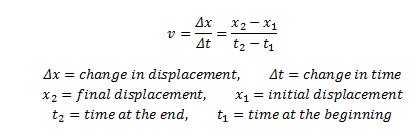v found using the above formula is the average velocity VAVG within the time Δt. When Δt becomes small (Δt→0), v becomes the instantaneous velocity.

Suppose the final displacement made was in two successive movements by the object in opposite directions (way forward and after backward). Then the above expression can be modified into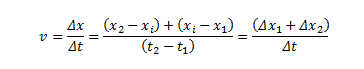Where xi is the displacement at the intermediate stage.

This result can be extended to any number of smaller motions.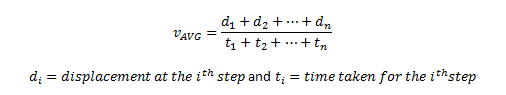Above results are independent of the acceleration experienced by the object. Now consider the motion of an object with constant acceleration.

If the acceleration is constant and the initial velocity is  v1 and final velocity is v2, then the average velocity is given by

##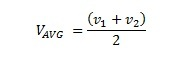How to Find Average Velocity – Examples

1. A car travels 2km to the north and then moves in the southward direction for another 4km. If the whole journey takes 15 mins, find the average velocity of the car during the journey. The journey was made in two parts. After the 15 minutes, the final displacement is 2km south of the starting point. Therefore, the average velocity is given by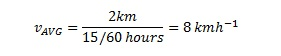1. A runner completes a 100m sprint in 9.8 seconds. Find the average velocity of the runner.
1. A train passes a station at 12kmh-1 and after an hour later passes another station. If the train constantly accelerated  and had velocity 48kmh-1 when passing the second station, what is the average velocity of the train within the hour?